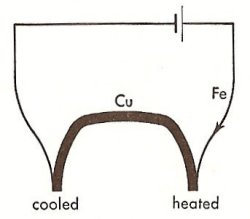A# Peltier effectPeltier effect. From Duckworth, Electricity & Magnetism, p 181.

The Peltier effect is one of three reversible thermoelectric phenomena (often known simply as thermoelectric effects), the others being the Seebeck effect and the Thomson effect. The Peltier effect is named after the French physicist Jean Charles Athanase Peltier (1785–1845), who discovered it in 1834 – eight years after Thomas Seebeck published his results on the first thermoelectric effect, which bears his name.

Peltier found that the junctions of dissimilar metals were heated or cooled, depending upon the direction in which an electrical current passed through them. Heat generated by current flowing in one direction was absorbed if the current was reversed. (Note that the effect always involves pairs of junctions, whether the junctions are explicit or just implied in the balance of the circuit.) The Peltier effect is found to be proportional to the first power of the current, not to its square, as is the irreversible generation of heat caused by resistance throughout the circuit.

As an example of the Peltier effect, consider the circuit shown here. Under these conditions it is observed, as indicated in the diagram, that the right-hand junction is heated, showing that electrical energy is being transformed into heat energy. Meanwhile, heat energy is transformed into electrical energy at the left junction, thereby causing it to be cooled. When the current is reversed, heat is absorbed at the right junction and produced at the left one.

Absorption or production of heat at a current-carrying junction is an indication that an electromotive force (emf) exists at the junction. Suppose the direction of the main current is the same as that produced by the junction emf, π. Each current carrier, q, is accelerated as it crosses the junction, gaining energy

W = π q.    (1)

This energy must be absorbed from the environment, in which event the junction is cooled. When the diagram above is interpreted in the light of this argument, it is seen that at each junction the copper is positive with respect to the iron.

The heat absorbed per second at a junction carrying a current I amperes is give by:

Heat absorbed/sec = πabI   (2)

where the current is from metal a to metal b. The junction emf, πab, is known as the Peltier coefficient; it is the heat energy absorbed per second at the junction per unit current, that is, the heat energy absorbed per unit charge. πab is positive if metal a is positive with respect to metal b (thus πCu, Fe is positive). The magnitude of πab is a function of the temperature of the junction. For identical temperatures πab = –πba. Thus, if the direction of the current in equation (2) is reversed, the heat absorbed per second is

Heat absorbed/sec = πbaI    (3)

which should be, and is, opposite in sign to equation (2).

Heat produced at a junction is a combination of Joule heat and "borrowed" heat contributed by connections elsewhere in the circuit, whether purposeful or not. Similarly, heat absorbed by a junction will be "pumped" into the other junctions distributed around the circuit. Semiconductor materials formulated especially for use in Peltier devices show "heat pumping" efficiency several times that of the most active metal pairings. These devices are used in applications ranging from lunch box coolers/warmers to laboratory instruments and communications systems. Quite large temperature differences can be generated by cascading or pyramiding Peltier devices such that each level in the pyramid acts as a heat sink for the level above.